#Function Repository Resource:

# CofactorMatrix (4.1.0)current version: 5.0.0 »

Give the matrix of cofactors for a given input matrix

Contributed by: Wolfram|Alpha Math Team
 ResourceFunction["CofactorMatrix"][mat] gives the cofactor matrix of the matrix mat.

## Details

The (i,j) cofactor of a square matrix is, up to a change in sign, equal to the determinant of the matrix obtained by deleting the ith row and jth column.

## Examples

### Basic Examples (4)

Find the matrix of cofactors of an input matrix:

 In:=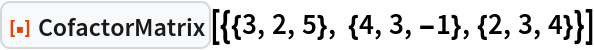Out=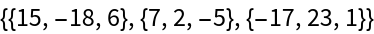The identity matrix is equal to its own cofactor matrix:

 In:=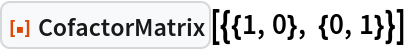Out=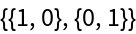Find the matrix of cofactors of another matrix:

 In:=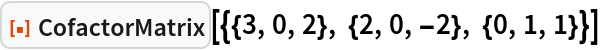Out=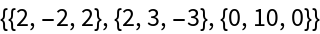Find the cofactor matrix of a matrix having symbolic elements:

 In:=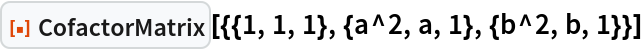Out=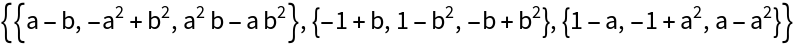### Scope (1)

CofactorMatrix returns unevaluated for non-square matrix input:

 In:=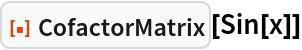Out=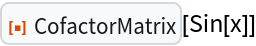In:=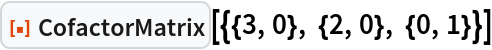Out=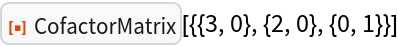### Properties and Relations (1)

The cofactor matrix is related to the Adjugate by transposition:

 In:=Out=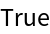## Publisher

Wolfram|Alpha Math Team

## Version History

• 5.0.0 – 23 March 2023
• 4.1.0 – 11 May 2021
• 4.0.0 – 24 January 2020
• 3.0.0 – 24 January 2020
• 2.0.0 – 06 September 2019
• 1.0.0 – 10 July 2019